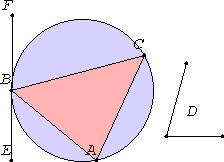# Proposition 34

From a given circle to cut off a segment admitting an angle equal to a given rectilinear angle.

Let ABC be the given circle, and the angle at D the given rectilinear angle.It is required to cut off from the circle ABC a segment admitting an angle equal to the given rectilinear angle, the angle at D.

Draw EF touching ABC at the point B. Construct the angle FBC equal to the angle at D on the straight line FB and at the point B on it.

III.32

Then, since a straight line EF touches the circle ABC, and BC has been drawn across from the point of contact at B, the angle FBC equals the angle constructed in the alternate segment BAC.

But the angle FBC equals the angle at D, therefore the angle in the segment BAC equals the angle at D.

Therefore from the given circle ABC the segment BAC has been cut off admitting an angle equal to the given rectilinear angle, the angle at D.

Q.E.F.

## Guide

This proposition is not used in the rest of the Elements.